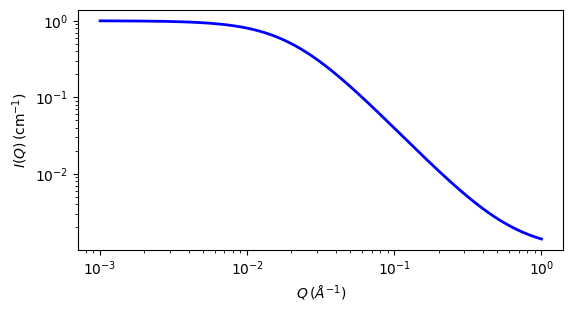# lorentz

Ornstein-Zernicke correlation length model

Parameter

Description

Units

Default value

scale

Scale factor or Volume fraction

None

1

background

Source background

cm-1

0.001

cor_length

Screening length

50

The returned value is scaled to units of cm-1 sr-1, absolute scale.

Lorentz (Ornstein-Zernicke Model)

Definition

The Ornstein-Zernicke model is defined by

$I(q)=\frac{\text{scale}}{1+(qL)^2}+\text{background}$

The parameter $$L$$ is the screening length cor_length.

For 2D data the scattering intensity is calculated in the same way as 1D, where the $$q$$ vector is defined as

$q=\sqrt{q_x^2 + q_y^2}$Fig. 109 1D plot corresponding to the default parameters of the model.

Source

lorentz.py

References

1. L.S. Qrnstein and F. Zernike, Proc. Acad. Sci. Amsterdam 17, 793 (1914), and Z. Phys. 19, 134 (1918), and 27, 761 {1926); referred to as QZ.

Authorship and Verification

• Author: